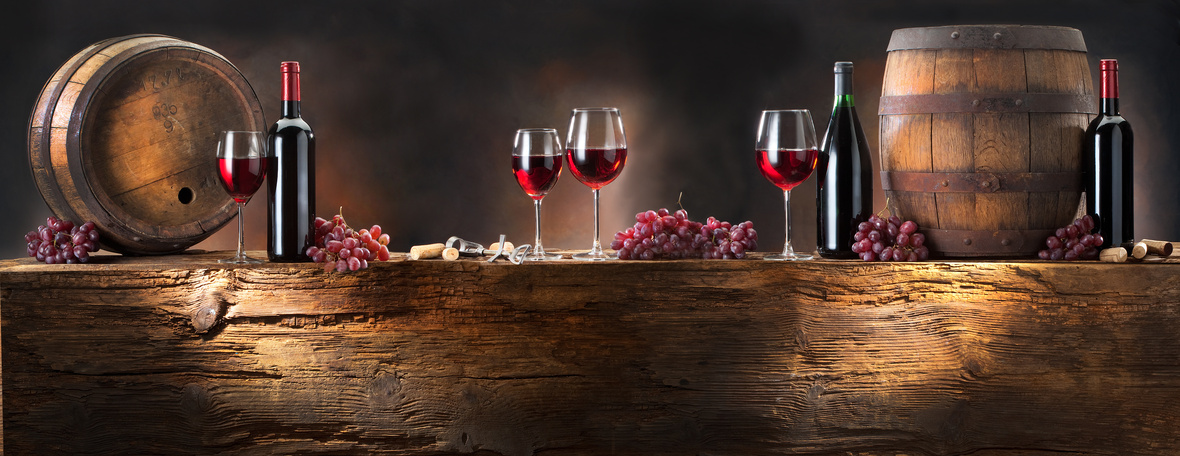# A Sneak Peak To Our Members-Only Society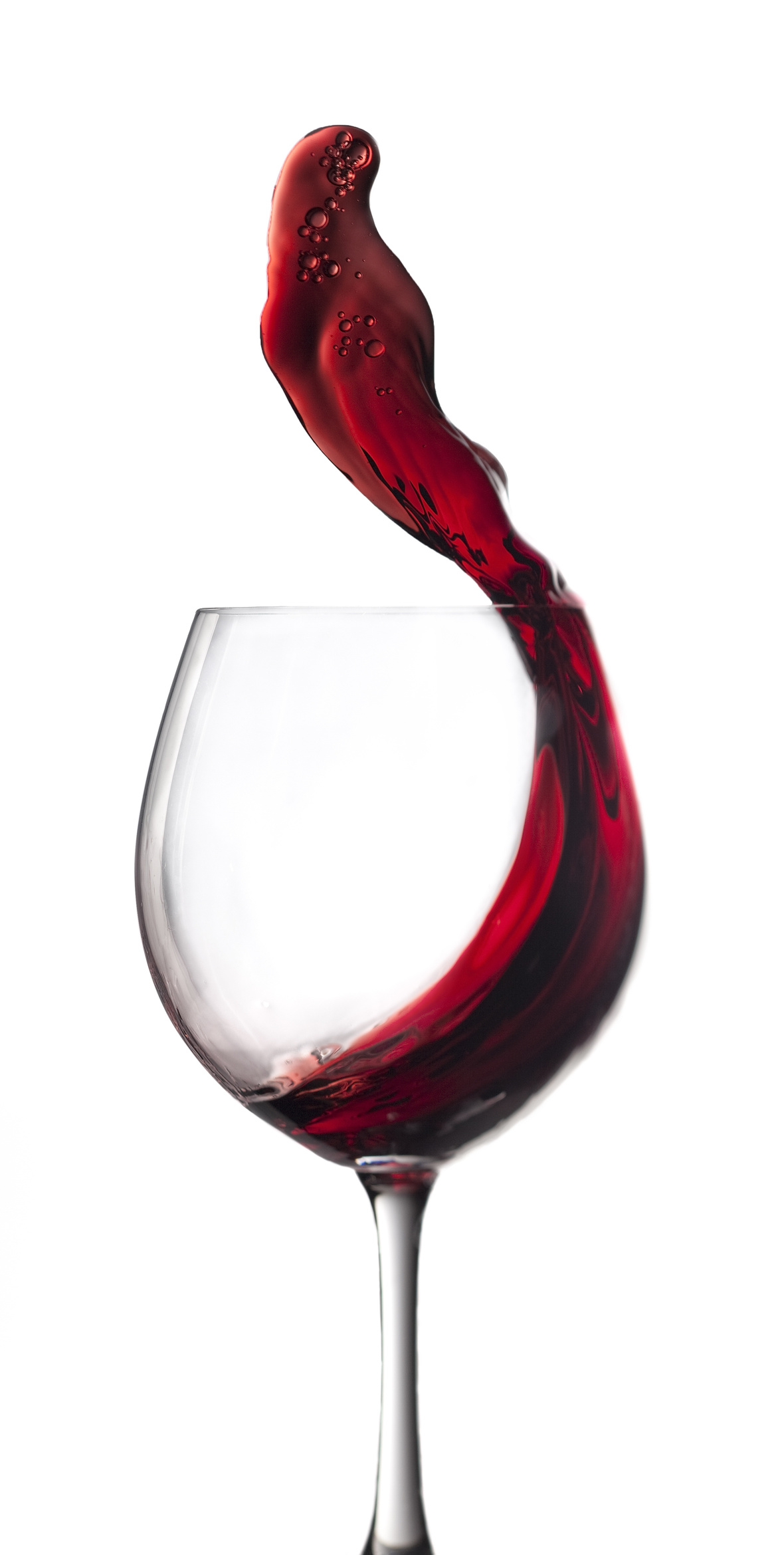Hi Wine Lover,

The Shall We Wine team is excited to share our monthly newsletter. Our goal is to introduce you to our favorite wines, winemakers, varietals, wine regions and places to buy and drink wine.

Cheers,

The Shall We Wine Team

 /* styles */

## SHALL WE WINE: THE MEMBERSHIP/* styles */ Shall We Wine Membership: The Ultimate Sip of Approval Host like a boss, dine like a boss… never miss a wine moment! Membership to Shall We Wine is our way of recognizing our most loyal wine tribe ⁠— the best way to access and take advantage of members-only perks. Shall We Wine membership is like one big soiree uniting beverage lovers far and wide. From novice to professional, we invite you to our society of fun! We offer 3 tiers of Shall We Wine membership: Cru (Community) Membership, Premier Cru Membership and ultimately, Grand Cru Membership. DETAILS COMING SOON!
 table div table+table+table+table+table+table+table+table div table{width:100%;padding:0}table div table+table+table+table+table+table+table+table div table img{width:96.23%;padding:0;float:none}table div table+table+table+table+table+table+table+table div table td{width:100%;padding:0 1.88% 18px}/* styles */## DISCOUNT OFFER FOR YOU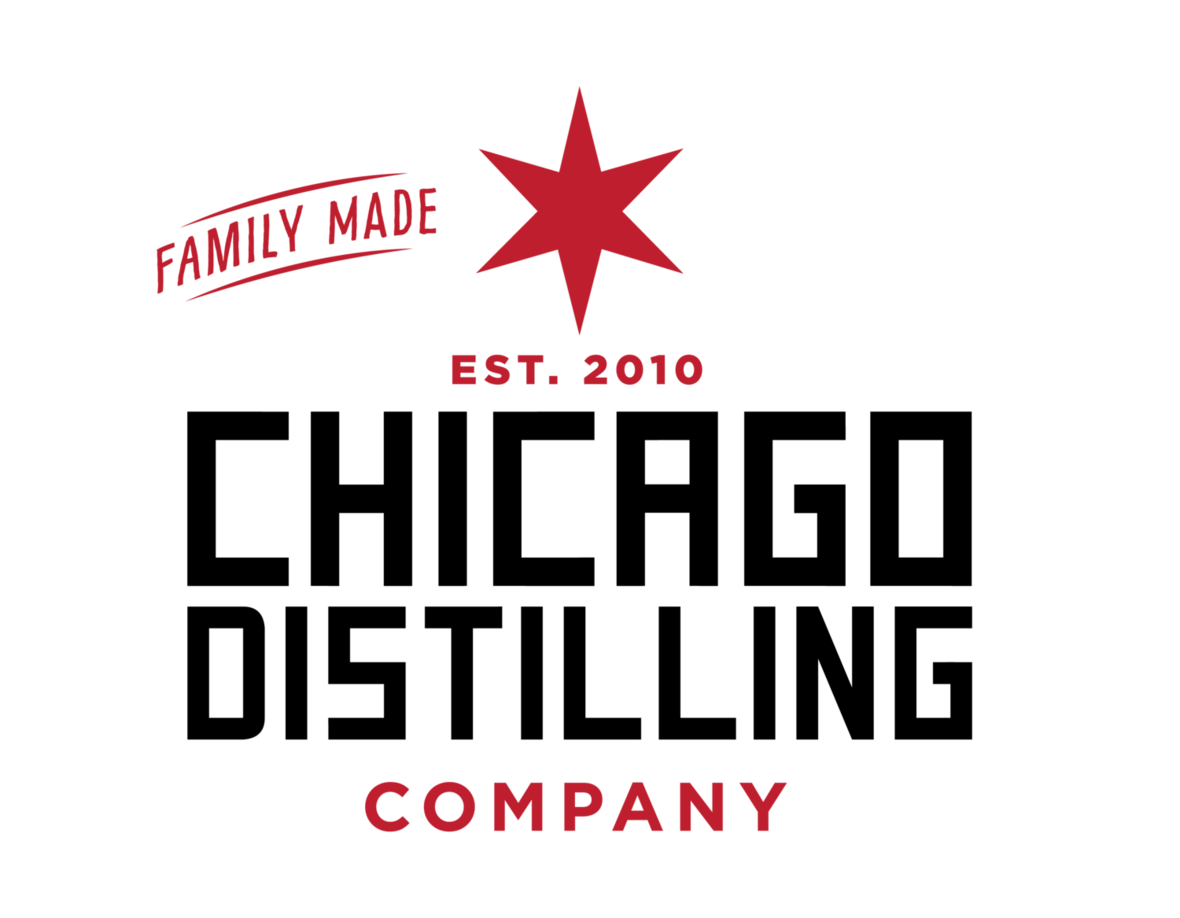Who doesn't love a discount?!

Our friends at *Chicago Distilling Company* are offering \$5 bottles of Finn's Gin. This gin and the owners were featured at our April 9, 2020 event.

Use code SWW5. This is for pickup only at their Chicago location.

 table div table+table+table+table+table+table+table+table+table+table+table div table{width:100%;padding:0}table div table+table+table+table+table+table+table+table+table+table+table div table img{width:96.23%;padding:0;float:none}table div table+table+table+table+table+table+table+table+table+table+table div table td{width:100%;padding:0 1.88% 18px}/* styles */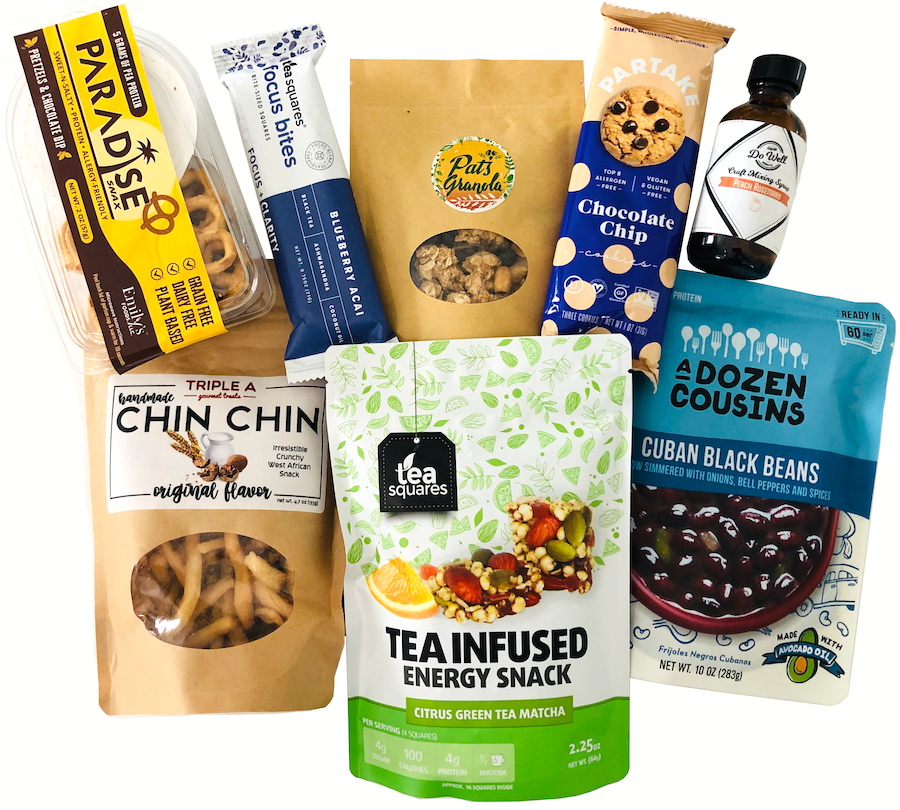August is National Black Business Month. Looking for a way to discover black-owned brands to support? Check out this newly launched discovery box!

GoodFoodBrands is highlighting black-owned food and beverage entrepreneurs who ventured out to start their own business and created amazingly delicious and healthy products. Discover your new favorite cookies, foods, snacks!

More at GoodFoodBrands.org

 table div table+table+table+table+table+table+table+table+table+table+table+table+table+table div table{width:100%;padding:0}table div table+table+table+table+table+table+table+table+table+table+table+table+table+table div table img{width:96.23%;padding:0;float:none}table div table+table+table+table+table+table+table+table+table+table+table+table+table+table div table td{width:100%;padding:0 1.88% 18px}/* styles */## ABC: ALWAYS BRING CHARDONNAY VIRTUAL EVENT

 /* styles */ On the cusp of the 25th Anniversary of the “ABC” movement, Anything But Chardonnay, and the New York Times article that sparked a decades-long debate, veteran California Chardonnay pioneer Randy Ullom from Kendall-Jackson, revisits the topic, and reinvents the ABC acronym: Always Bring Chardonnay! Who: David Ramey of Ramey Wine Cellars, and Niki Wente of Wente Vineyards join Randy and Moderator Karen MacNeil for A LIVE Virtual Tasting What (THE WINES): 2018 Kendall-Jackson Vintner’s Reserve Chardonnay, SRP \$17 – www.kj.com 2017 Ramey Russian River Valley Chardonnay, SRP \$42 – www.rameywine.com 2018 Wente Vineyards Riva Ranch Vineyard Chardonnay, SRP \$22 – www.wentevineyards.com If you are unable to locate at your local shop, all these wines are also available for purchase on www.wine.com. When: Thursday, August 20, 5:30 p.m. PACIFIC COST: Complimentary to participate! Register via Zoom link below.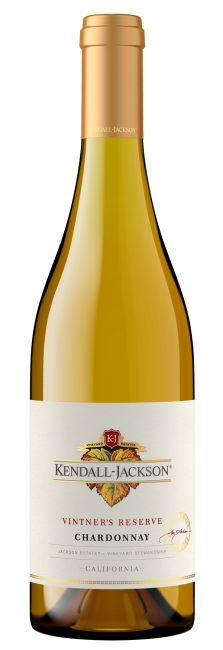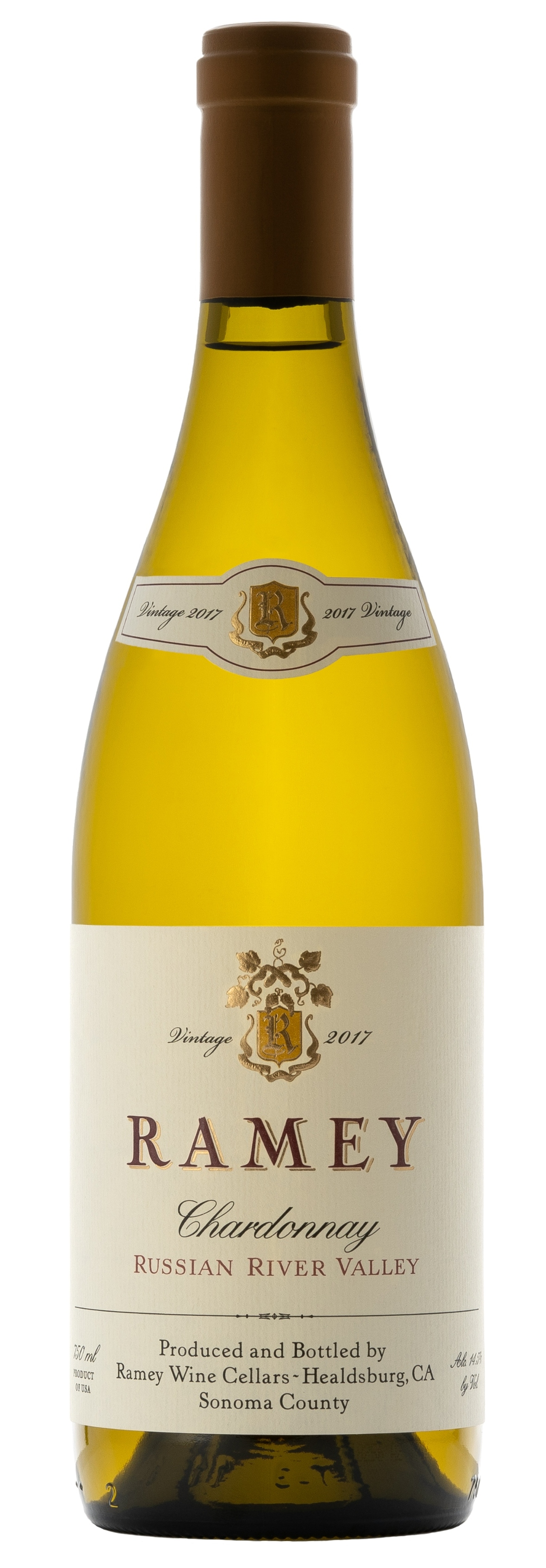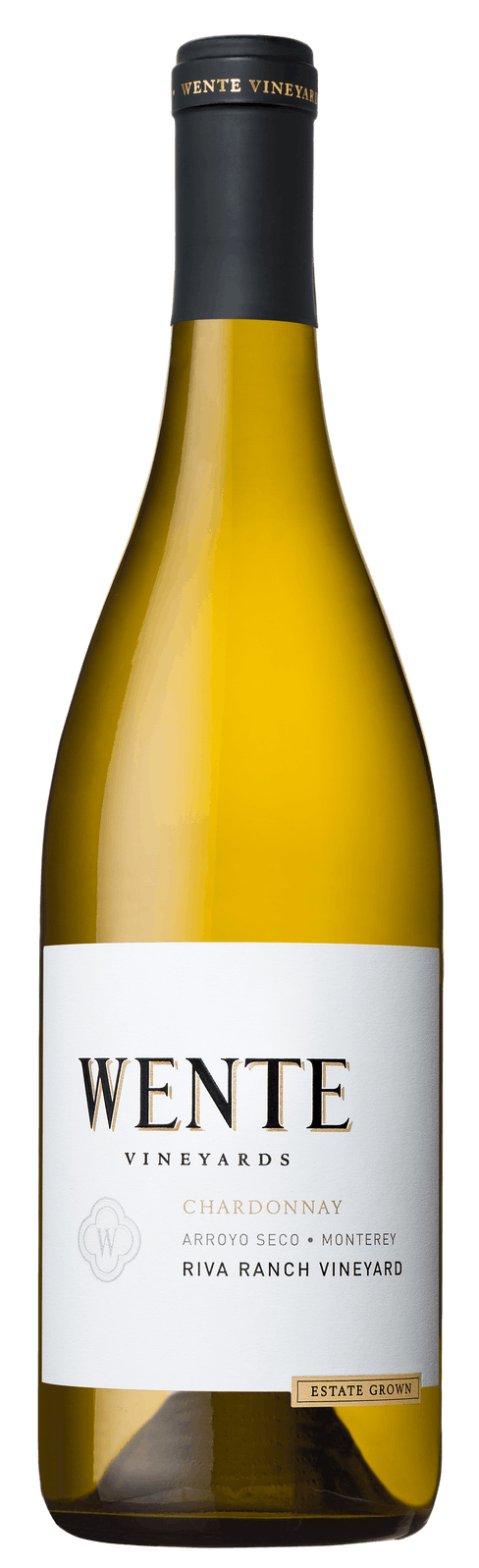table div table+table+table+table+table+table+table+table+table+table+table+table+table+table+table+table+table+table+table+table div table{width:100%;padding:0}table div table+table+table+table+table+table+table+table+table+table+table+table+table+table+table+table+table+table+table+table div table img{width:96.23%;padding:0;float:none}table div table+table+table+table+table+table+table+table+table+table+table+table+table+table+table+table+table+table+table+table div table td{width:100%;padding:0 1.88% 18px}/* styles */## SPOTLIGHT: WOMEN IN WINE - ESTHER PINUAGA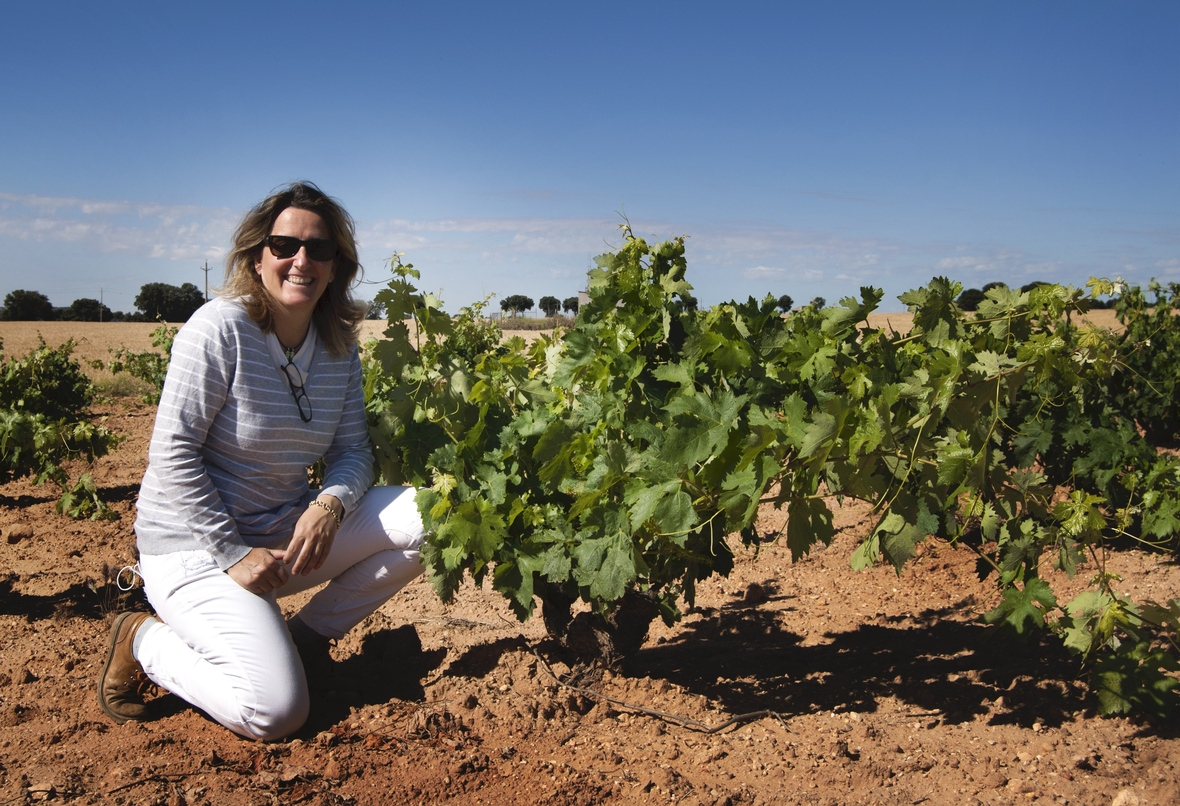Esther Pinuaga left a career as an international business consultant in the early 2000's to return to the estate founded by her father. A remarkable woman, Esther not only manages the sales and business side of the winery business, she is also the President of the Spanish Organic Winegrowers Association.

## SPOTLIGHT: WOMEN IN WINE - BRENAE ROYALBrenae's career in agriculture stemmed directly from hours of enjoyment planting flowers and picking weeds alongside her grandmother. She soon began raising pigs and joined Future Farmers of America (FFA).

By 2015, Brenae was named Ranch Manager for Monte Rosso Vineyard, an iconic property where grapes are grown for the likes of Mount Peak Winery. “When I started, it was intimidating. Here I am this 24-year-old coming in to manage Monte Rosso, a world-renowned vineyard and one of the oldest in the state. It’s difficult and complex but I love it,” she said.

 table div table+table+table+table+table+table+table+table+table+table+table+table+table+table+table+table+table+table+table+table+table+table+table+table+table div table{width:100%;padding:0}table div table+table+table+table+table+table+table+table+table+table+table+table+table+table+table+table+table+table+table+table+table+table+table+table+table div table img{width:96.23%;padding:0;float:none}table div table+table+table+table+table+table+table+table+table+table+table+table+table+table+table+table+table+table+table+table+table+table+table+table+table div table td{width:100%;padding:0 1.88% 18px}/* styles */## ARTICLE FEATURE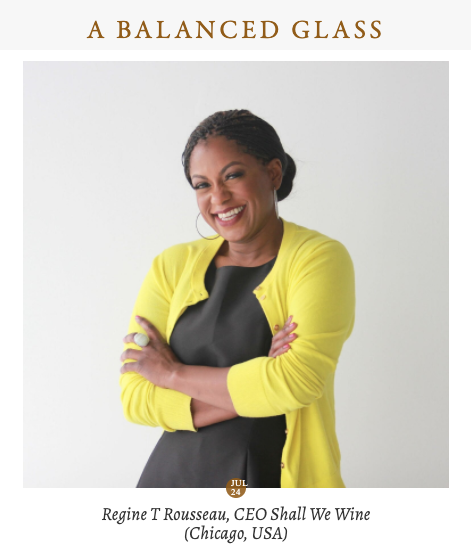Featured in A Balanced Glass. We talk Shall We Wine, the beverage industry, and the rewards and challenges of being an entrepreneur!

Check it out here!

 table div table+table+table+table+table+table+table+table+table+table+table+table+table+table+table+table+table+table+table+table+table+table+table+table+table+table+table+table div table{width:100%;padding:0}table div table+table+table+table+table+table+table+table+table+table+table+table+table+table+table+table+table+table+table+table+table+table+table+table+table+table+table+table div table img{width:96.23%;padding:0;float:none}table div table+table+table+table+table+table+table+table+table+table+table+table+table+table+table+table+table+table+table+table+table+table+table+table+table+table+table+table div table td{width:100%;padding:0 1.88% 18px}/* styles */## ENGAGING READS FOR THE MONTHLiquor.com

These Black Women In Wine Are Redefining the Industry
The Zoe Report

Celebrity Rosé
Wine Enthusiast

 table div table+table+table+table+table+table+table+table+table+table+table+table+table+table+table+table+table+table+table+table+table+table+table+table+table+table+table+table+table+table+table div table{width:100%;padding:0}table div table+table+table+table+table+table+table+table+table+table+table+table+table+table+table+table+table+table+table+table+table+table+table+table+table+table+table+table+table+table+table div table img{width:96.23%;padding:0;float:none}table div table+table+table+table+table+table+table+table+table+table+table+table+table+table+table+table+table+table+table+table+table+table+table+table+table+table+table+table+table+table+table div table td{width:100%;padding:0 1.88% 18px}/* styles */It is incredibly moving to receive random notes of love! Below is from a fellow reader of Searching for Cloves & Lilies:

"Your book has blown me away. It is magnificent.. from the soul of you.. so deep and un self conscious. I got it this afternoon and read it from cover to cover. I need a second round to fully absorb the intensity. Mighty powerful! Thanks for printing and making available... not to mention creating!" Fondly, Lestable div table+table+table+table+table+table+table+table+table+table+table+table+table+table+table+table+table+table+table+table+table+table+table+table+table+table+table+table+table+table+table+table+table+table+table+table div table{width:100%;padding:0}table div table+table+table+table+table+table+table+table+table+table+table+table+table+table+table+table+table+table+table+table+table+table+table+table+table+table+table+table+table+table+table+table+table+table+table+table div table img{width:96.23%;padding:0;float:none}table div table+table+table+table+table+table+table+table+table+table+table+table+table+table+table+table+table+table+table+table+table+table+table+table+table+table+table+table+table+table+table+table+table+table+table+table div table td{width:100%;padding:0 1.88% 18px}/* styles */## BOOK A PRIVATE OR CORPORATE COCKTAIL HOUR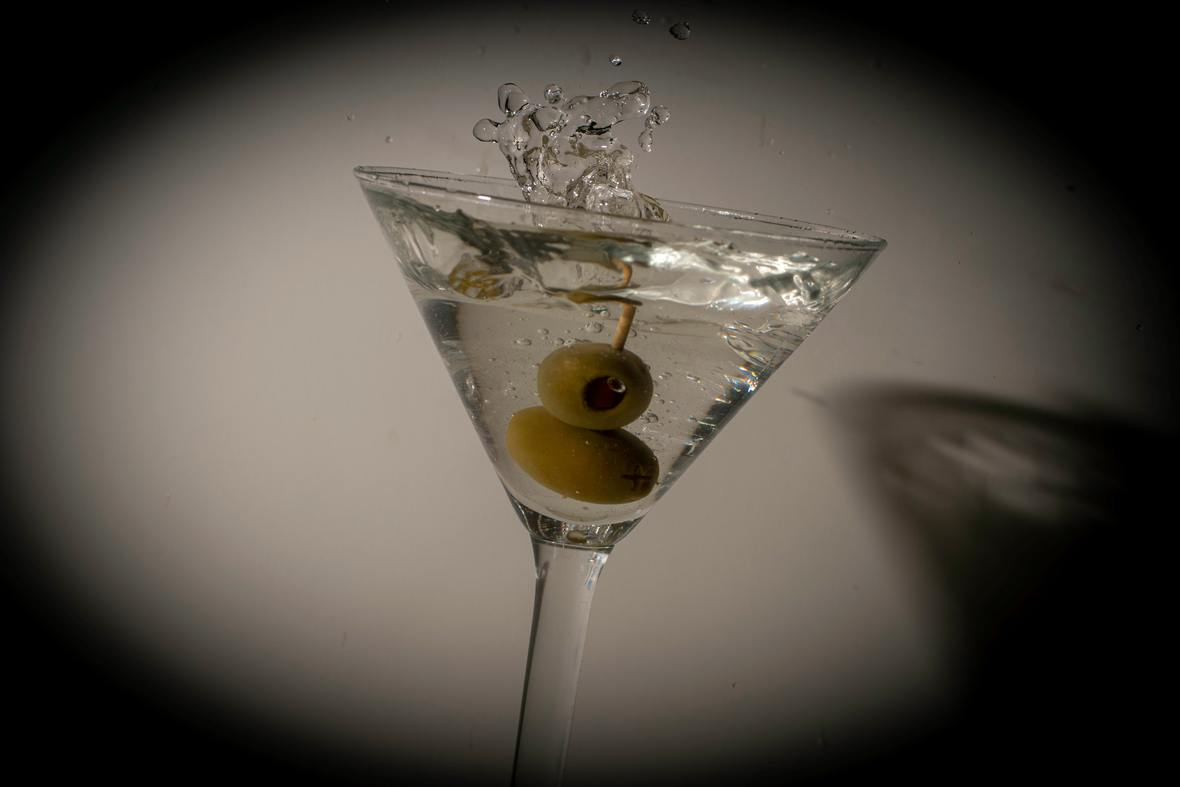Maybe you want to host a cocktail hour for a small group of friends.

Or maybe you want to throw a cocktail hour to celebrate a birthday or special milestone.

Or maybe you're tired of hosting the same kind of virtual events for your team and are looking for something to make them different and engage them.

Whatever the case is, you've come to the right place. Just as Shall We Wine does in-person experiences, we also plan experiences for corporate virtual events. We work with our corporate clients to create wine and spirit-focused experiences!

 table div table+table+table+table+table+table+table+table+table+table+table+table+table+table+table+table+table+table+table+table+table+table+table+table+table+table+table+table+table+table+table+table+table+table+table+table+table+table+table+table div table{width:100%;padding:0}table div table+table+table+table+table+table+table+table+table+table+table+table+table+table+table+table+table+table+table+table+table+table+table+table+table+table+table+table+table+table+table+table+table+table+table+table+table+table+table+table div table img{width:96.23%;padding:0;float:none}table div table+table+table+table+table+table+table+table+table+table+table+table+table+table+table+table+table+table+table+table+table+table+table+table+table+table+table+table+table+table+table+table+table+table+table+table+table+table+table+table div table td{width:100%;padding:0 1.88% 18px}/* styles */## DID YOU KNOW...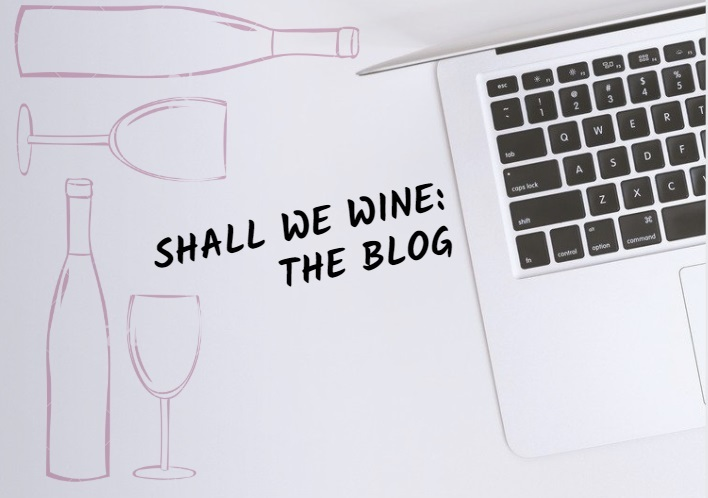We have a blog! Here you will find things like our Wine of the Week and other special wine & spirits topics! Stay in the know and read up on some of the cool things we've been sharing.

 table div table+table+table+table+table+table+table+table+table+table+table+table+table+table+table+table+table+table+table+table+table+table+table+table+table+table+table+table+table+table+table+table+table+table+table+table+table+table+table+table+table+table+table div table{width:100%;padding:0}table div table+table+table+table+table+table+table+table+table+table+table+table+table+table+table+table+table+table+table+table+table+table+table+table+table+table+table+table+table+table+table+table+table+table+table+table+table+table+table+table+table+table+table div table img{width:96.23%;padding:0;float:none}table div table+table+table+table+table+table+table+table+table+table+table+table+table+table+table+table+table+table+table+table+table+table+table+table+table+table+table+table+table+table+table+table+table+table+table+table+table+table+table+table+table+table+table div table td{width:100%;padding:0 1.88% 18px}/* styles *//* styles */ CLICK BELOW
 table div table+table+table+table+table+table+table+table+table+table+table+table+table+table+table+table+table+table+table+table+table+table+table+table+table+table+table+table+table+table+table+table+table+table+table+table+table+table+table+table+table+table+table+table+table+table div table{width:100%;padding:0}table div table+table+table+table+table+table+table+table+table+table+table+table+table+table+table+table+table+table+table+table+table+table+table+table+table+table+table+table+table+table+table+table+table+table+table+table+table+table+table+table+table+table+table+table+table+table div table table{padding:0;float:left!important;width:26.059%!important}table div table+table+table+table+table+table+table+table+table+table+table+table+table+table+table+table+table+table+table+table+table+table+table+table+table+table+table+table+table+table+table+table+table+table+table+table+table+table+table+table+table+table+table+table+table+table div table td{padding-left:29px;padding-right:29px}table div table+table+table+table+table+table+table+table+table+table+table+table+table+table+table+table+table+table+table+table+table+table+table+table+table+table+table+table+table+table+table+table+table+table+table+table+table+table+table+table+table+table+table+table+table+table div table table+table,table div table+table+table+table+table+table+table+table+table+table+table+table+table+table+table+table+table+table+table+table+table+table+table+table+table+table+table+table+table+table+table+table+table+table+table+table+table+table+table+table+table+table+table+table+table+table div table table+table+table{float:left!important;width:26.059%!important}table div table+table+table+table+table+table+table+table+table+table+table+table+table+table+table+table+table+table+table+table+table+table+table+table+table+table+table+table+table+table+table+table+table+table+table+table+table+table+table+table+table+table+table+table+table+table div table table td,table div table+table+table+table+table+table+table+table+table+table+table+table+table+table+table+table+table+table+table+table+table+table+table+table+table+table+table+table+table+table+table+table+table+table+table+table+table+table+table+table+table+table+table+table+table+table div table table+table td,table div table+table+table+table+table+table+table+table+table+table+table+table+table+table+table+table+table+table+table+table+table+table+table+table+table+table+table+table+table+table+table+table+table+table+table+table+table+table+table+table+table+table+table+table+table+table div table table+table+table td{padding-left:0;padding-right:20px}table div table+table+table+table+table+table+table+table+table+table+table+table+table+table+table+table+table+table+table+table+table+table+table+table+table+table+table+table+table+table+table+table+table+table+table+table+table+table+table+table+table+table+table+table+table+table div table table+table+table+table{float:left!important;width:21.822999999999993%!important}table div table+table+table+table+table+table+table+table+table+table+table+table+table+table+table+table+table+table+table+table+table+table+table+table+table+table+table+table+table+table+table+table+table+table+table+table+table+table+table+table+table+table+table+table+table+table div table table+table+table+table td{padding-left:0;padding-right:0}/* styles */
 table div table+table+table+table+table+table+table+table+table+table+table+table+table+table+table+table+table+table+table+table+table+table+table+table+table+table+table+table+table+table+table+table+table+table+table+table+table+table+table+table+table+table+table+table+table+table+table div table{width:100%;padding:0}table div table+table+table+table+table+table+table+table+table+table+table+table+table+table+table+table+table+table+table+table+table+table+table+table+table+table+table+table+table+table+table+table+table+table+table+table+table+table+table+table+table+table+table+table+table+table+table div table img{width:96.23%;padding:0;float:none}table div table+table+table+table+table+table+table+table+table+table+table+table+table+table+table+table+table+table+table+table+table+table+table+table+table+table+table+table+table+table+table+table+table+table+table+table+table+table+table+table+table+table+table+table+table+table+table div table td{width:100%;padding:0 1.88% 18px}/* styles */## THANKS AND TOGETHERNESSIn an effort to maintain social distancing measures, Shall We Wine will continue to devote our time to connecting with each and every one of our friends and supporters like you virtually. We're constantly sharing fun content to help brighten up your weeks!

We appreciate your patience and cooperation as we work through these times together. Thank you for your continued support in making Shall We Wine your go-to wine & lifestyle resource!/* styles */ Learn, Drink, Repeat! The Shall We Wine Team
 Like   Tweet   Pin   +1   in